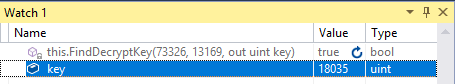in the past days I wondered why some parts of the encryption and decryption keys for the network packet encryption (aka SimpleModulus) are actually the same. So I tried to dig a bit in the SimpleModulus algorithm and the usage of these keys.

Lets see how this keys (e.g. S->C) look like...

Encryption Key: 73326, 109989, 98843, 171058, 13169, 19036, 35482, 29587, 62004, 64409, 35374, 64599
Decryption Key: 73326, 109989, 98843, 171058, 18035, 30340, 24701, 11141, 62004, 64409, 35374, 64599

These keys are consisting of twelve 32-bit integers. What can you see is, that the first and the last 4 integers are identical for the encryption and decryption key. Additionally these keys use almost only 16 bits - only the first 4 numbers are actually over it. One of these keys is used by the server to encrypt outgoing network packets, and the other is used by the client to decrypt incoming network packets. So it's some kind of a private/public key pair.

So, I had a closer look at the encryption code which uses one of these keys:

``````this.RingBuffer = ((keys ^ this.CryptBuffer) * keys) % keys;
this.RingBuffer = ((keys ^ (this.CryptBuffer ^ (this.RingBuffer & 0xFFFF))) * keys) % keys;
this.RingBuffer = ((keys ^ (this.CryptBuffer ^ (this.RingBuffer & 0xFFFF))) * keys) % keys;
this.RingBuffer = ((keys ^ (this.CryptBuffer ^ (this.RingBuffer & 0xFFFF))) * keys) % keys;
``````

What you can see here is, that the index of 8-11 is used for a XOR-Operation (so we call it XOR-Key x), the index 4-7 is used for a multiplication (Encryption-Key k) and the first index of 0-3 is used for a modulus operation (Modulus-Key m). Because each encryption key uses at most only 16 bits, there are 4 chained 16-bit encryptions, which looks like it's easy to break just with a bruteforce attack.

The decryption code looks similar:

``````this.CryptBuffer = (ushort)(keys ^ ((this.RingBuffer * keys) % keys));
this.CryptBuffer = (ushort)(keys ^ ((this.RingBuffer * keys) % keys) ^ (this.RingBuffer & 0xFFFF));
this.CryptBuffer = (ushort)(keys ^ ((this.RingBuffer * keys) % keys) ^ (this.RingBuffer & 0xFFFF));
this.CryptBuffer = (ushort)(keys ^ ((this.RingBuffer * keys) % keys) ^ (this.RingBuffer & 0xFFFF));
``````

For the sake of simplification, we can ignore the xor-key as it plays no role, because it's the same for both:

encrypt(inputdec ) -> inputdec * kenc (mod m)

decrypt(inputenc ) -> inputenc * kdec (mod m)

This encryption is based on modular multiplicative inverses.
One requirement, that this encryption can work like this at all, is that k and m are always coprime - which is the case for all our example key values.

With this knowledge we can build the following formula:

kenc * kdec (mod m) = 1

So when we know one of the two keys, we can calculate the other one.

Did I forget to mention Webzen didn't even try hard to hide these keys? The game client has two files with them in it's data folder, Enc1.dat and Dec2.dat - of course this files are encrypted by another weak XOR-Encryption :-)

#### Example:

13169 * kdec (mod 73326) = 1

To solve this we could use the extended euclidean algorithm but our computer is also fast enough to find kdec with the slower naive algorithm which tests every possible value of kdec from 0 to (m-1):

``````private bool FindDecryptKey(uint modulusKey, uint encryptKey, out uint decryptKey)
{
decryptKey = 0;
for (uint i = 0; i < modulusKey; i++)
{
if (encryptKey * i % modulusKey == 1)
{
decryptKey = i;
return true;
}
}

return false;
}
``````

When we call it with our example values, we get:We can also use the same function to get the encryption key for a known decryption key - so this encryption algorithm is totally insecure.

#### Conclusion

To protect the network packets from the prying eyes of a hacker, this encryption algorithm is not good enough. Anyone who wants to run a MU online server in production should really use something else.

My little Webzen rant: Not without reason, security experts say to not roll your own crypto - Webzen developers have seemed to ignore this advice. Not long after they introduced it (somewhere around 2003~2004), hacks were popping up and server developers had reverse engineered the encryption algorithm. However, Webzen didn't respond accordingly - they kept using it at official game servers until the end of 2011 when the version of ex700 arrived. No wonder, the servers were full of cheaters ;-)

##### What this means for my project?

At the moment, the OpenMU.Network project only contains the keys which are used on the server side. I thought that would be a good idea from keeping hackers away, but it also limits the usage of the project for other kind of applications (e.g. event bots run by server owners or for MU server development tools like network MITM proxies). So, I will extend the project by the other part of the keys (and a key generator, of course) soon.
As I gained some better understanding of the algorithm I'll also try to make the code more understandable.

Long term, I also think about adding the subsequent encryption layer which was introduced with version of ex700, aka "Packet Twister", which source code is also already (partially) known to the private server development community.